• RNN网络 RNN模型计算分为两步，第一步，计算第ｔ个时间步的隐藏层ａ；第二步，计算t步的预测值ｙ。 其中Wax和Waa两组参数分别与前一层的激活a和当前数据x结合，也可将其合二为一，并与x和a的连接计算...公式中的Γ
RNN网络

RNN模型计算分为两步，第一步，计算第ｔ个时间步的隐藏层ａ；第二步，计算t步的预测值ｙ。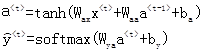其中Wax和Waa两组参数分别与前一层的激活a和当前数据x结合，也可将其合二为一，并与x和a的连接计算。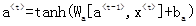在RNN网络中最常用激活函数是tanh，有时也用ReLU，一般只在最后一层使用sigmoid或softmax。

LSTM网络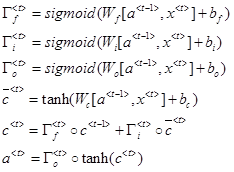相对于基础的RNN，LSTM增加了c（Cell）状态单元，可将其看做在计算激活a的过程中保存的当前状态。它与a都在工作过程中一直向下一步传递。

公式中的Γ表示门控gate，门控使用sigmoid函数计算得出0-1之间的值，用于过滤（保留或去掉）数据。LSTM中增加的门控分别是：遗忘门f，输入门i和输出门o，它们分别控制在更新当前cell内容时，之前的cell占比，当前的cell占比，以及如何用cell生成ａ。

举个简单的例子：用LSTM生成文字，当输入“回车”时，说明本段已结束，之前的内容被遗忘门丢弃；当前输入是“笑脸”时，输入本身没太大意义，被输入门丢弃；如果之前输入了“本段没”，当前时间步又输入了“用”，加在一起是“本段没用”，则输出门将其丢弃。（请领会精神）。

一般RNN的层数不会特别多（三层就差不多了），除了层数以外，还需要按时间步向后传播，计算量也很大。门控值由前一激活层a和当前时间步的数据x计算得出，每一门控分别对应一组参数w和b，因此，参数比RNN大三倍。这与Attention注意力机制非常相似，用当前输入和当前状态计算权值，给数据流加权。

GRU网络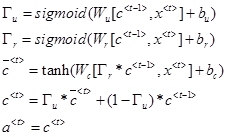GRU可视为LSTM的变体，它使用了两个门控，更新门u控制当前状态和之前状态的占比（LSTM使用输入门和遗忘门实现）；去掉了输出门，用状态值作为激活值，简化算法结构；另外加入了重置门r，用于控制当状状态对前一状态的依赖程度。

深度神经网络一般都面临梯度爆炸和梯度消失的问题，梯度爆炸问题可以通过加入归一化层或梯度修剪的方式解决，LSTM和GRU主要用于缓解梯度消失问题，如GRU中当更新门趋近0时，当前状态与之前状态相等，类似于用残差网络解决梯度消失问题。它使RNN网络能在更长的时间步中工作，并支持更深层次的网络。

双向网络

双向网络在求y时，需要计算向前的激活层和向后的激活层，常用解决类似于完型填空的问题，需要考虑上下文的场景。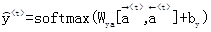展开全文• 深度学习——循环神经网络GRU公式推导 0、注意 在整篇的文章中，无论是输入的X向量，还是隐藏层得到的S向量，这些都是列向量 1、从RNN到GRU 在之前的文章中，我们具体推导了循环神经网络RNN的前向和反向传播过程，...
深度学习——循环神经网络GRU公式推导
0、注意
在整篇的文章中，无论是输入的X向量，还是隐藏层得到的S向量，这些都是列向量
1、从RNN到GRU
在之前的文章中，我们具体推导了循环神经网络RNN的前向和反向传播过程，具体细节可以参考深度学习——循环神经网络RNN公式推导这篇文章。下面，我们开始介绍RNN的一个变形结构GRU神经网络。
我们首先简单的回顾一下RNN神经网络的结构，以及一个RNN隐藏层神经元的基本结构。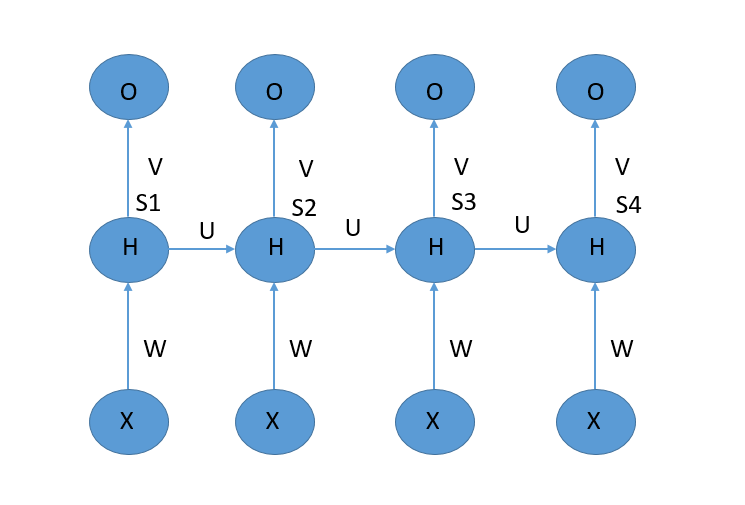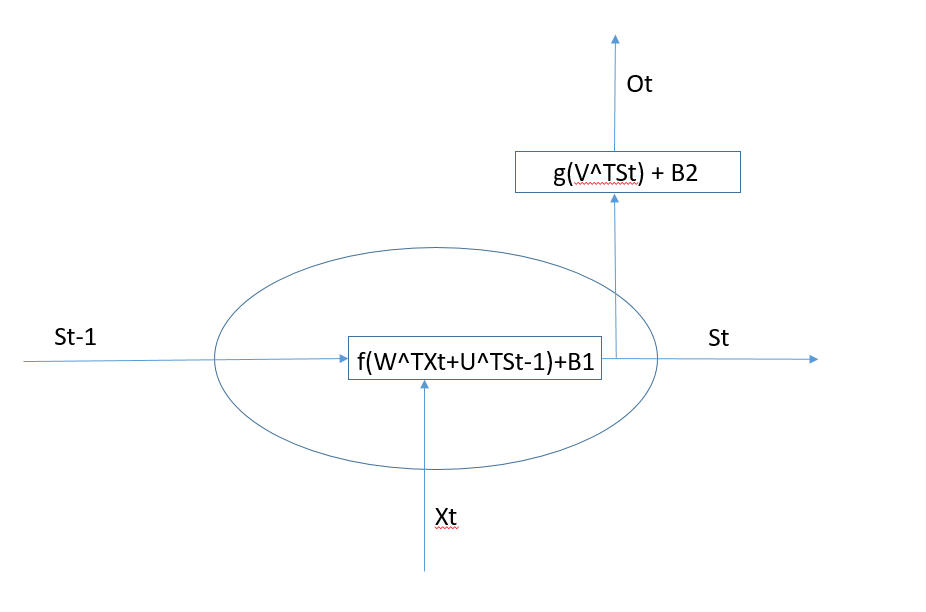上面的两张图是RNN神经网络整体的结构图和一个隐藏层神经单元的结构图。
1.1 RNN神经网络的局限性-梯度消失与爆炸问题
如果在训练的过程中发生了梯度消失的问题，会导致我们的权重无法被更新，最终导致训练失败。如果发生了梯度爆炸的问题，意味着梯度过大，从而大幅度的更新网络参数，造成了网络的不稳定。最终导致网络的不稳定。在极端的情况下，权重的值变得特别大，以至于结果会溢出。
在RNN神经网络，随着时间t的推移，使得网络的长度越来越大，导致在反向传播的过程中容易发生梯度消失或者梯度爆炸。这也就是说，在RNN网络中，当前的预测需要用到比较久远的信息的时候，会因为梯度消失或者梯度爆炸的原因，会引起长期依赖的问题。
那么，如何解决这种长期依赖的问题呢？
一种比较好的方法是引入门控机制来控制信息的累积速度，包括有选择的加入新的信息，有选择的遗忘之前积累的信息等等。这一类网络称为基于门控的循环神经网络，常见的网络类型包括长短期记忆网络LSTM和门控循环网络GRU。本篇文章中，我们先来介绍GRU神经网络。
2 GRU神经网络
2.1 网络结构介绍
GRU神经网络的整体结构与RNN神经网络的整体结构相同，区别在于GRU神经网络中的隐藏层单元引入了门控单元，其具体的结构如下图所示：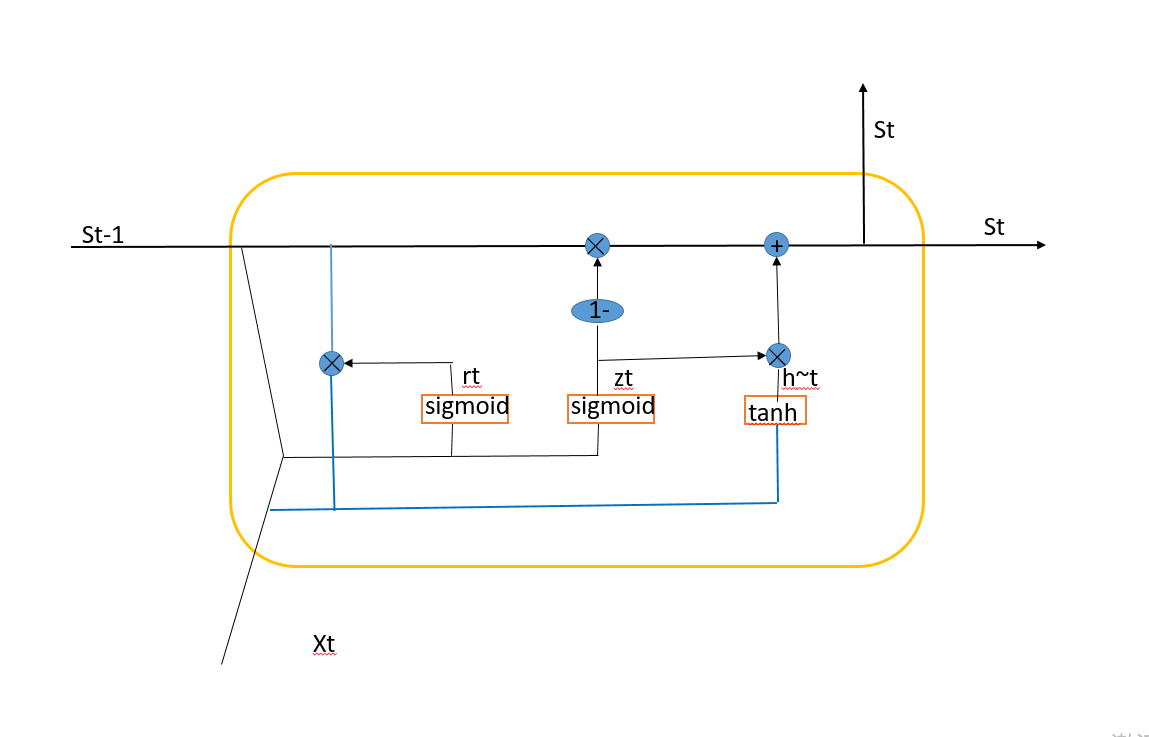相比于RNN神经元，GRU的神经元略微的有些复杂，下面，逐个的对上面的计算进行说明。

在获得前一个时刻神经单元的输入$S_{t-1}$和当前时刻的输入$X_t$之后，首先是将两者进行合并输入到r(t)中去。计算过程为：
$net_r(t)=W_r^TX_t+U_r^TS_{t-1}+B_r$
$r(t )= sigmoid(net_r(t))$
下一个过程是将前一个时刻神经单元的输入$S_{t-1}$和当前时刻的输入$X_t$输入到$z(t)$中去，具体计算过程为：
$net_z(t)=W_z^TX_t+U_z^TS_{t-1}+B_z$
$z_t = sigmoid(net_z(t))$
再然后是产生hht的部分，这一部分相对于其他两个部分复杂一点，其输入包括当前时刻的输入$X_t$和计算出来的$r(t)$, 首先是计算出来的$r(t)$和$S_{t-1}$进行对位相乘，再将计算结果与$X_t$进行加法合并。
$net_{hh}(t)=W_{hh}^TX_t+U_{hh}^T(r(t)*S_{t-1})+B_{hh}$
$hh(t)=tanh(net_{hh}(t))$
在计算完成$r(t),z(t),hh(t)$之后，我们要计算的就是该单元的输出值以及向输出层传递的值。先计算该单元的输出值：
$S_t=(1-z(t))*S_{t-1}+z(t )* hh(t)$
最后计算输出值(如果该单元有输出值)：
$net_o(t)=W_o^TS_t+B_o$
$o(t)=sigmoid(net_o(t))$

2.2 传递参数介绍
根据上面的计算过程，我们可以提炼出整个GRU结构所需要的权重矩阵的个数。对于$r(t)$位置，其需要两个权重矩阵$W_r,U_r$。对于$z(t)$位置，其需要两个权重矩阵$W_z,U_z$，对于$hh(t)$，其需要两个权重矩阵$W_{hh},U_{hh}$。最后对于输出层，有一个权重矩阵$W_o$。
$r(t)=>[W_r,U_r,B_r]$，$z(t)=>[W_z,U_z,B_z]$，$hh(t)=>[W_{hh},U_{hh},B_{hh}]$，$o(t)=>[W_o,B_o]$。
2.3 前向传播过程
我们之前已经介绍了计算过程，其计算过程也可以看做是前向传播过程。所以，这里我们给出一些代码来实现一下前向传播的过程(过程也可以参考纯Python和PyTorch对比实现门控循环单元GRU及反向传播这篇文章,这篇文章中的hh(t)的计算与本文稍有不同)：
#encoding=utf-8
import numpy as np

def sigmoid(x):
return 1/(1 + np.exp(-x))
def tanh(x):

class GRUCell:
def __init__(self,W_r,W_z,W_hh,U_r,U_z,U_hh,W_o,br,bz,bh,bo):
self.W_r = W_r
self.W_z = W_z
self.W_hh = W_hh
self.U_r = U_r
self.U_z = U_z
self.U_hh = U_hh
self.W_o = W_o
self.br = br
self.bz = bz
self.bh = bh
self.bo = bo

def forward(self,X,S_prev):
net_rt = np.dot(self.W_r.T,X)+np.dot(self.U_r,S_prev) + br
rt = sigmoid(net_rt)
net_zt = np.dot(self.W_z.T,X)+ np.dot(self.U_z,S_prev) + bz
zt = sigmoid(net_zt)
net_hht = np.dot(self.W_hh.T,X) + np.dot(self.U_hh.T,(rt * S_prev) + bh)
hht = np.tanh(net_hht)
St = (1 - zt) * S_prev + z(t) * hht
net_ot = np.dot(self.W_o.T,St) + bo
Ot = sigmoid(net_ot)
return net_rt,rt,net_zt,zt,net_hht,hht,St,net_ot,Ot

2.4 反向传播算法
2.5.1 误差函数
这里，我选用的softmax函数交叉熵的误差计算，其中交叉熵的计算公式为：
$J=-∑_{i=1}^Ly_{ri}ln(y_i)$
其中$y_{ri}$是指真实标签值的第i个属性，$y_i$表示预测值的第i个属性，对于真实的标签$y_r$和预测输出的属性值$y$的属性的个数为L。
softmax的计算公式为：
$y_i=\frac{e^{o_i}}{∑_{j=1}^Le^{o_j}}$
其中，$o_i$表示输出层计算出来向量o中的第i个属性。
$\frac{∂J}{∂o_i} = y_{i} - y_{ri}$
$\frac{∂J}{∂o}= y-y_r$
求导的具体过程可以参考我之前的文章深度学习——损失函数与梯度推导。
2.5.2 误差关于nethh(t)的梯度计算
$\frac{∂J}{∂net_{hh}(t)}=\frac{∂J}{∂S_t}*\frac{∂S_t}{∂hh(t)}*\frac{∂hh(t)}{∂net_{hh}(t)}=\frac{∂J}{∂S_t}*z(t)*\frac{∂hh(t)}{∂net_{hh}(t)}$
由已知得到，$hh(t)=tanh(net_{hh}(t))$，以及tanh的导数，则有:
$δ_{net_{hh}}(t)=\frac{∂J}{∂net_{hh}(t)}=\frac{∂J}{∂S_t}*z(t)*(1 - {hh}(t)^2)$
2.5.4 误差关于netz(t)的梯度计算
$\frac{∂J}{∂net_{z}(t)}=\frac{∂J}{∂S_t}*S_{t-1}*(-1)*\frac{∂z(t)}{∂net_z(t)}+\frac{∂J}{∂S_t}*hh(t)*\frac{∂z(t)}{∂net_z(t)}$
根据已知得到$z(t)=sigmoid(net_{z}(t))$，以及sigmoid的导数，则有：
$δ_{net_{z}}(t)=\frac{∂J}{∂net_{z}(t)}=\frac{∂J}{∂S_t}[hh(t)-S_{t-1}]*z(t)*(1-z(t))$
2.5.5 误差关于netr(t)的梯度计算
$\frac{∂J}{∂net_{r}(t)}=\frac{∂J}{∂S_t}*\frac{∂J}{∂hh(t)}*\frac{∂hh(t)}{∂r(t)}*\frac{∂r(t)}{∂net_r(t)}=\frac{∂J}{∂hh(t)}*\frac{∂hh(t)}{∂net_{hh}(t)}*\frac{∂net_{hh}(t)}{∂r(t)}*\frac{∂r(t)}{∂net_r(t)}$
根据已知 $net_{hh}(t)=W_{hh}^TX_t+U_{hh}^T(r(t)*S_{t-1})$，以及上面的推导，有：
$\frac{∂net_{hh}(t)}{∂r(t)}=U_{hh}S_{t-1}$
又有，$r(t)=sigmoid(net_{r}(t))$，则原式子可以求导为：
$δ_{net_{r}}(t)=\frac{∂L}{∂net_{r}(t)}=\frac{∂L}{∂net_{hh}(t)}*(U_{hh}S_{t-1})*r(t)*(1-r(t))$
$δ_{net_{r}}(t)=\frac{∂L}{∂net_{r}(t)}=δ_{net_{hh}}(t)*(U_{hh}S_{t-1})*r(t)*(1-r(t))$
2.5.6 误差关于St-1的梯度运算
$\frac{∂J}{∂S_{t-1}}=\frac{∂J}{∂S_{t}}*[(1-z(t))+\frac{∂S_t}{∂z(t)}*\frac{∂z(t)}{∂S_{t-1}}+\frac{∂S_t}{∂hh(t)}\frac{∂hh(t)}{∂S_{t-1}}]$
其中
$\frac{∂z(t)}{∂S_{t-1}}=\frac{∂z(t)}{∂net_z(t)}*\frac{∂net_{z(t)}}{∂S_{t-1}}=U_z\frac{∂z(t)}{∂net_z(t)}$
其次
$\frac{∂hh(t)}{∂S_{t-1}}=\frac{∂hh(t)}{∂net_{hh}(t)}\frac{∂net_{hh}(t)}{∂S_{t-1}}$
其中：
$\frac{∂net_{hh}(t)}{∂S_{t-1}}=U_{hh}r(t)+\frac{∂net_{hh}(t)}{∂r(t)}*\frac{∂r(t)}{∂net_r(t)}*\frac{∂net_r(t)}{∂S_{t-1}}=U_{hh}r(t)+U_r[\frac{∂net_{hh}(t)}{∂r(t)}*\frac{∂r(t)}{∂net_r(t)}]$
将上述计算公式进行合并有：
$\frac{∂J}{∂S_{t-1}}=\frac{∂J}{∂S_{t}}*[(1-z(t))+U_z\frac{∂S_t}{∂z(t)}\frac{∂z(t)}{∂net_z(t)}+\frac{∂S_t}{∂hh(t)}\frac{∂hh(t)}{∂net_{hh}(t)}(U_{hh}r(t)+U_r[\frac{∂net_{hh}(t)}{∂r(t)}*\frac{∂r(t)}{∂net_r(t)}])]$
则，原式最终等于：
$δ_{S_{t-1}}=\frac{∂J}{∂S_{t-1}}=\frac{∂J}{∂S_{t}}*(1-z(t))+(U_zδ_{net_z}(t)+U_{hh}(δ_{net_{hh}}(t)*r(t))+(U_rδ_{net_t}(t))$
2.6 delta计算总结
$δ_{net_{hh}}(t)=\frac{∂J}{∂net_{hh}(t)}=\frac{∂J}{∂S_t}*z(t)*(1 - {hh}(t)^2)$
$δ_{net_{z}}(t)=\frac{∂J}{∂net_{z}(t)}=\frac{∂J}{∂S_t}[hh(t)-S_{t-1}]*z(t)*(1-z(t))$
$δ_{net_{r}}(t)=\frac{∂L}{∂net_{r}(t)}=δ_{net_{hh}}(t)*(U_{hh}S_{t-1})*r(t)*(1-r(t))$
$δ_{S_{t-1}}=\frac{∂J}{∂S_{t-1}}=\frac{∂J}{∂S_{t}}*(1-z(t))+(U_zδ_{net_z}(t)+U_{hh}(δ_{net_{hh}}(t)*r(t))+(U_rδ_{net_t}(t))$
2.7、权重参数梯度
根据我们最初的设定，我们需要更新的包括$W_r,U_r，W_z,U_z，W_{hh},U_{hh}$。六个权重矩阵。
2.6.1 对于Wr权重矩阵的求导
回顾$W_r$出现的公式：
$net_r(t)=W_r^TX_t+U_r^TS_{t-1}+B_r$
容易就得到：
$\frac{∂J}{∂W_{r}}=X_tδ_{net_r}(t)^T$
2.6.2 对于Ur权重的求导
回顾$U_r$出现的公式：
$net_r(t)=W_r^TX_t+U_r^TS_{t-1}+B_r$
容易就得到：
$\frac{∂J}{∂U_{r}}=S_{t-1}δ_{net_r}(t)^T$
2.6.3 对于Br的求导
回顾$B_r$出现的公式：
$net_r(t)=W_r^TX_t+U_r^TS_{t-1}+B_r$
容易就得到：
$\frac{∂J}{∂U_{r}}=δ_{net_r}(t)$
2.6.4 对于Wz权重的求导
回顾$W_z$出现的公式：
$net_z(t)=W_z^TX_t+U_z^TS_{t-1}+B_z$
容易得到：
$\frac{∂J}{∂W_{z}}=X_tδ_{net_z}(t)^T$
2.6.5 对于Uz权重的求导
回顾$U_z$出现的公式：
$net_z(t)=W_z^TX_t+U_z^TS_{t-1}+B_z$
容易得到：
$\frac{∂J}{∂U_{z}}=S_{t-1}δ_{net_z}(t)^T$
2.6.6 对于Bz的求导
回顾$B_z$出现的公式：
$net_z(t)=W_z^TX_t+U_z^TS_{t-1}+B_z$
容易得到：
$\frac{∂J}{∂B_{z}}=δ_{net_z}(t)$
2.6.7 对于Whh权重的求导
回顾$W_{hh}$出现的公式：
$net_{hh}(t)=W_{hh}^TX_t+U_{hh}^T(r(t)*S_{t-1})+B_{hh}$
容易得到：
$\frac{∂J}{∂W_{hh}}=X_tδ_{net_{hh}}(t)^T$
2.6.8 对于Uhh权重的求导
回顾$U_{hh}$出现的公式：
$net_{hh}(t)=W_{hh}^TX_t+U_{hh}^T(r(t)*S_{t-1})+B_{hh}$
容易得到：
$\frac{∂J}{∂U_{hh}}=(r(t)*S_{t-1})δ_{net_{hh}}^T$
2.6.9 对于Bhh的求导
回顾$B_{hh}$出现的公式：
$net_{hh}(t)=W_{hh}^TX_t+U_{hh}^T(r(t)*S_{t-1})+B_{hh}$
容易得到：
$\frac{∂J}{∂B_{hh}}=δ_{net_{hh}}$
2.7 权重更新
根据上述推导过程，我们确定了每一个时刻$W_r,W_z,W_{hh},U_r,U_z,U_{hh},B_r,B_z,B_{hh}$的导数，下面我们在更新的时候，需要将所有的时刻的导数进行累加。则最终更新公式为：
$W_{r}(new)=W_r - α∑_{t=1}^TW_r$
$W_{z}(new)=W_z - α∑_{t=1}^TW_z$
$W_{hh}(new)=W_{hh} - α∑_{t=1}^TW_{hh}$
$U_{r}(new)=U_r - α∑_{t=1}^TU_r$
$U_{z}(new)=U_z - α∑_{t=1}^TU_z$
$U_{hh}(new)=U_{hh} - α∑_{t=1}^TU_{hh}$
$B_{r}(new)=B_r - α∑_{t=1}^TB_r$
$B_{z}(new)=B_z - α∑_{t=1}^TB_z$
$B_{hh}(new)=B_{hh} - α∑_{t=1}^TB_{hh}$
2.8 对于输出中的Wo和Bo的更新
我们先回顾一下$net_o$
$net_o(t)=W_o^TS_t+B_o$
在此之前，我们已经计算出来了，误差J关于$o$的梯度：
$\frac{∂J}{∂o}= y-y_r$
则误差J关于$net_o$的梯度为：
$δ_{net_o}=\frac{∂J}{∂net_o}=\frac{∂J}{∂o}*f'(o)=( y-y_r)*f'(o)$
则对于$W_o$的梯度为：
$\frac{∂J}{∂W_o}=Oδ_{net_o}^T$
对于$B_o$的梯度为：
$\frac{∂J}{∂B_o}=δ_{net_o}$
其中f是$net_o$所采用的激活函数。
在设计GRU网络的时候，可能在最后的时刻才会有输出，此时，对于Wo，Bo的更新不需要按照时刻进行累加的过程。如果每一个时刻都有输出，则需要进行累加之后，进行更新。


展开全文• 文章目录循环神经网络门控循环单元（GRU）门控循环单元重置门和更新门候选隐藏状态隐藏状态输出结果长短期记忆（LSTM）输入门、遗忘门和输出门候选记忆细胞记忆细胞隐藏状态输出结果深度循环神经网络双向循环神经...
文章目录循环神经网络门控循环单元（GRU）门控循环单元重置门和更新门候选隐藏状态隐藏状态输出结果长短期记忆（LSTM）输入门、遗忘门和输出门候选记忆细胞记忆细胞隐藏状态输出结果深度循环神经网络双向循环神经网络
循环神经网络
在循环神经网络中输入数据是存在时间相关性的，也就是说，前一个时间点的数据会对后一时间点的数据产生影响。假设 $\boldsymbol{X}_t \in \mathbb{R}^{n \times d}$ 是序列中时间步 $t$ 的小批量输入，$\boldsymbol{H}_t \in \mathbb{R}^{n \times h}$ 是该时间步的隐藏变量。与多层感知机不同的是，这里我们保存上一时间步的隐藏变量 $\boldsymbol{H}_{t-1}$，并引入一个新的权重参数 $\boldsymbol{W}_{hh} \in \mathbb{R}^{h \times h}$，该参数用来描述在当前时间步如何使用上一时间步的隐藏变量。具体来说，时间步 $t$ 的隐藏变量的计算由当前时间步的输入和上一时间步的隐藏变量共同决定：
$\boldsymbol{H}_t = \phi(\boldsymbol{X}_t \boldsymbol{W}_{xh} + \boldsymbol{H}_{t-1} \boldsymbol{W}_{hh} + \boldsymbol{b}_h).$
与多层感知机相比，我们在这里添加了 $\boldsymbol{H}_{t-1} \boldsymbol{W}_{hh}$ 一项。由上式中相邻时间步的隐藏变量 $\boldsymbol{H}_t$ 和 $\boldsymbol{H}_{t-1}$ 之间的关系可知，这里的隐藏变量能够捕捉截至当前时间步的序列的历史信息，就像是神经网络当前时间步的状态或记忆一样。因此，该隐藏变量也称为隐藏状态。隐藏状态中 $\boldsymbol{X}_t \boldsymbol{W}_{xh} + \boldsymbol{H}_{t-1} \boldsymbol{W}_{hh}$ 的计算等价于 $\boldsymbol{X}_t$与$\boldsymbol{H}_{t-1}$ 连结后的矩阵乘以$\boldsymbol{W}_{xh}$与$\boldsymbol{W}_{hh}$连结后的矩阵。由于隐藏状态在当前时间步的定义使用了上一时间步的隐藏状态，上式的计算是循环的。使用循环计算的网络即循环神经网络（recurrent neural network）。
循环神经网络有很多种不同的构造方法。含上式所定义的隐藏状态的循环神经网络是极为常见的一种。在时间步 $t$，输出层的输出和多层感知机中的计算类似：
$\boldsymbol{O}_t = \boldsymbol{H}_t \boldsymbol{W}_{hq} + \boldsymbol{b}_q.$
循环神经网络的参数包括隐藏层的权重 $\boldsymbol{W}_{xh} \in \mathbb{R}^{d \times h}$、$\boldsymbol{W}_{hh} \in \mathbb{R}^{h \times h}$ 和偏差 $\boldsymbol{b}_h \in \mathbb{R}^{1 \times h}$，以及输出层的权重 $\boldsymbol{W}_{hq} \in \mathbb{R}^{h \times q}$ 和偏差 $\boldsymbol{b}_q \in \mathbb{R}^{1 \times q}$。值得一提的是，即便在不同时间步，循环神经网络也始终使用这些模型参数。因此，循环神经网络模型参数的数量不随时间步的增加而增长。上图展示了循环神经网络在3个相邻时间步的计算逻辑。在时间步 $t$，隐藏状态的计算可以看成是将输入 $\boldsymbol{X}_t$ 和前一时间步隐藏状态 $\boldsymbol{H}_{t-1}$ 连结后输入一个激活函数为 $\phi$ 的全连接层。该全连接层的输出就是当前时间步的隐藏状态 $\boldsymbol{H}_t$，且模型参数为 $\boldsymbol{W}_{xh}$ 与 $\boldsymbol{W}_{hh}$ 的连结，偏差为 $\boldsymbol{b}_h$。当前时间步 $t$ 的隐藏状态 $\boldsymbol{H}_t$ 将参与下一个时间步 $t+1$ 的隐藏状态 $\boldsymbol{H}_{t+1}$ 的计算，并输入到当前时间步的全连接输出层。
门控循环单元（GRU）
循环神经网络中如何通过时间反向传播？一文中介绍了循环神经网络中的梯度计算方法。我们发现，当时间步数较大或者时间步较小时，循环神经网络的梯度较容易出现衰减或爆炸。虽然裁剪梯度可以应对梯度爆炸，但无法解决梯度衰减的问题。通常由于这个原因，循环神经网络在实际中较难捕捉时间序列中时间步距离较大的依赖关系。
门控循环单元
门控循环神经网络（gated recurrent neural network）的提出，正是为了更好地捕捉时间序列中时间步距离较大的依赖关系。它通过可以学习的门来控制信息的流动。其中，门控循环单元（gated recurrent unit，GRU）是一种常用的门控循环神经网络。
重置门和更新门
如下图所示，门控循环单元中的重置门和更新门的输入均为当前时间步输入 $\boldsymbol{X}_t$ 与上一时间步隐藏状态 $\boldsymbol{H}_{t-1}$，输出由激活函数为 sigmoid 函数的全连接层计算得到。具体来说，假设样本数为 $n$、输入个数为 $d$（即每个样本包含的元素数，一般为词典大小）、隐藏单元个数为 $h$，给定时间步 $t$ 的小批量输入 $\boldsymbol{X}_t \in \mathbb{R}^{n \times d}$ 和上一时间步隐藏状态 $\boldsymbol{H}_{t-1} \in \mathbb{R}^{n \times h}$。重置门 $\boldsymbol{R}_t \in \mathbb{R}^{n \times h}$ 和更新门 $\boldsymbol{Z}_t \in \mathbb{R}^{n \times h}$ 的计算如下：
$\boldsymbol{R}_t = \sigma(\boldsymbol{X}_t \boldsymbol{W}_{xr} + \boldsymbol{H}_{t-1} \boldsymbol{W}_{hr} + \boldsymbol{b}_r)$
$\boldsymbol{Z}_t = \sigma(\boldsymbol{X}_t \boldsymbol{W}_{xz} + \boldsymbol{H}_{t-1} \boldsymbol{W}_{hz} + \boldsymbol{b}_z)$
其中 $\boldsymbol{W}_{xr}, \boldsymbol{W}_{xz} \in \mathbb{R}^{d \times h}$ 和 $\boldsymbol{W}_{hr}, \boldsymbol{W}_{hz} \in \mathbb{R}^{h \times h}$ 是权重参数，$\boldsymbol{b}_r, \boldsymbol{b}_z \in \mathbb{R}^{1 \times h}$ 是偏差参数。sigmoid 函数可以将元素的值变换到0和1之间。因此，重置门 $\boldsymbol{R}_t$ 和更新门 $\boldsymbol{Z}_t$ 中每个元素的值域都是$[0, 1]$。
候选隐藏状态
接下来，门控循环单元将计算候选隐藏状态来辅助稍后的隐藏状态计算。如下图所示，我们将当前时间步重置门的输出与上一时间步隐藏状态做按元素乘法（符号为$\odot$）。如果重置门中元素值接近0，那么意味着重置对应隐藏状态元素为0，即丢弃上一时间步的隐藏状态。如果元素值接近1，那么表示保留上一时间步的隐藏状态。然后，将按元素乘法的结果与当前时间步的输入连结，再通过含激活函数 tanh 的全连接层计算出候选隐藏状态，其所有元素的值域为$[-1, 1]$。具体来说，时间步 $t$ 的候选隐藏状态 $\tilde{\boldsymbol{H}}_t \in \mathbb{R}^{n \times h}$ 的计算为：
$\tilde{\boldsymbol{H}}_t = \text{tanh}(\boldsymbol{X}_t \boldsymbol{W}_{xh} + \left(\boldsymbol{R}_t \odot \boldsymbol{H}_{t-1}\right) \boldsymbol{W}_{hh} + \boldsymbol{b}_h)$
其中 $\boldsymbol{W}_{xh} \in \mathbb{R}^{d \times h}$ 和 $\boldsymbol{W}_{hh} \in \mathbb{R}^{h \times h}$ 是权重参数，$\boldsymbol{b}_h \in \mathbb{R}^{1 \times h}$ 是偏差参数。从上面这个公式可以看出，重置门控制了上一时间步的隐藏状态如何流入当前时间步的候选隐藏状态。而上一时间步的隐藏状态可能包含了时间序列截至上一时间步的全部历史信息。因此，重置门可以用来丢弃与预测无关的历史信息从而有助于捕捉时间序列里短期的依赖关系
隐藏状态
最后，时间步 $t$ 的隐藏状态 $\boldsymbol{H}_t \in \mathbb{R}^{n \times h}$ 的计算使用当前时间步的更新门 $\boldsymbol{Z}_t$ 来对上一时间步的隐藏状态 $\boldsymbol{H}_{t-1}$ 和当前时间步的候选隐藏状态 $\tilde{\boldsymbol{H}}_t$ 做组合：
$\boldsymbol{H}_t = \boldsymbol{Z}_t \odot \boldsymbol{H}_{t-1} + (1 - \boldsymbol{Z}_t) \odot \tilde{\boldsymbol{H}}_t$值得注意的是，更新门可以控制隐藏状态应该如何被包含当前时间步信息的候选隐藏状态所更新，如上图所示。假设更新门在时间步 $t'$ 到 $t$（$t' < t$）之间一直近似于1。那么，在时间步 $t'$ 到 $t$ 之间的输入信息几乎没有流入时间步 $t$ 的隐藏状态 $\boldsymbol{H}_t$。实际上，这可以看作是较早时刻的隐藏状态 $\boldsymbol{H}_{t'-1}$ 一直通过时间保存并传递至当前时间步 $t$。这个设计可以应对循环神经网络中的梯度衰减问题，并更好地捕捉时间序列中时间步距离较大的依赖关系（即长期的依赖关系）。
输出结果
将上面得到的隐藏状态 $H_t$ 输入神经网络，得到结果 $\boldsymbol{Y}_t \in \mathbb{R}^{n \times q}$：
$\boldsymbol{Y}_t = \boldsymbol{H}_t \boldsymbol{W}_{hq} + \boldsymbol{b}_q$
总结来说：

重置门有助于捕捉时间序列里短期的依赖关系；
更新门有助于捕捉时间序列里长期的依赖关系。

长短期记忆（LSTM）
LSTM 中引入了3个门，即输入门（input gate）、遗忘门（forget gate）和输出门（output gate），以及与隐藏状态形状相同的记忆细胞（某些文献把记忆细胞当成一种特殊的隐藏状态），从而记录额外的信息。
输入门、遗忘门和输出门
与门控循环单元中的重置门和更新门一样，如下图所示，长短期记忆的门的输入均为当前时间步输入$\boldsymbol{X}_t$ 与上一时间步隐藏状态 $\boldsymbol{H}_{t-1}$，输出由激活函数为 sigmoid 函数的全连接层计算得到。如此一来，这3个门元素的值域均为$[0,1]$。具体来说，假设样本数为 $n$，输入个数为 $d$，隐藏单元个数为 $h$，给定时间步 $t$ 的小批量输入 $\boldsymbol{X}_t \in \mathbb{R}^{n \times d}$ 和上一时间步隐藏状态 $\boldsymbol{H}_{t-1} \in \mathbb{R}^{n \times h}$。 时间步 $t$ 的输入门 $\boldsymbol{I}_t \in \mathbb{R}^{n \times h}$、遗忘门 $\boldsymbol{F}_t \in \mathbb{R}^{n \times h}$ 和输出门 $\boldsymbol{O}_t \in \mathbb{R}^{n \times h}$ 分别计算如下：
$\boldsymbol{I}_t = \sigma(\boldsymbol{X}_t \boldsymbol{W}_{xi} + \boldsymbol{H}_{t-1} \boldsymbol{W}_{hi} + \boldsymbol{b}_i)$
$\boldsymbol{F}_t = \sigma(\boldsymbol{X}_t \boldsymbol{W}_{xf} + \boldsymbol{H}_{t-1} \boldsymbol{W}_{hf} + \boldsymbol{b}_f)$
$\boldsymbol{O}_t = \sigma(\boldsymbol{X}_t \boldsymbol{W}_{xo} + \boldsymbol{H}_{t-1} \boldsymbol{W}_{ho} + \boldsymbol{b}_o)$
其中的 $\boldsymbol{W}_{xi}, \boldsymbol{W}_{xf}, \boldsymbol{W}_{xo} \in \mathbb{R}^{d \times h}$ 和 $\boldsymbol{W}_{hi}, \boldsymbol{W}_{hf}, \boldsymbol{W}_{ho} \in \mathbb{R}^{h \times h}$ 是权重参数，$\boldsymbol{b}_i, \boldsymbol{b}_f, \boldsymbol{b}_o \in \mathbb{R}^{1 \times h}$ 是偏差参数。
候选记忆细胞
接下来，长短期记忆需要计算候选记忆细胞 $\tilde{\boldsymbol{C}}_t$。它的计算与上面介绍的3个门类似，但使用了值域在$[-1, 1]$的 tanh 函数作为激活函数，如下图所示。具体来说，时间步 $t$ 的候选记忆细胞 $\tilde{\boldsymbol{C}}_t \in \mathbb{R}^{n \times h}$ 的计算为
$\tilde{\boldsymbol{C}}_t = \text{tanh}(\boldsymbol{X}_t \boldsymbol{W}_{xc} + \boldsymbol{H}_{t-1} \boldsymbol{W}_{hc} + \boldsymbol{b}_c)$
其中 $\boldsymbol{W}_{xc} \in \mathbb{R}^{d \times h}$ 和 $\boldsymbol{W}_{hc} \in \mathbb{R}^{h \times h}$ 是权重参数，$\boldsymbol{b}_c \in \mathbb{R}^{1 \times h}$ 是偏差参数。
记忆细胞
我们可以通过元素值域在$[0, 1]$的输入门、遗忘门和输出门来控制隐藏状态中信息的流动，这一般也是通过使用按元素乘法（符号为$\odot$）来实现的。当前时间步记忆细胞 $\boldsymbol{C}_t \in \mathbb{R}^{n \times h}$ 的计算组合了上一时间步记忆细胞和当前时间步候选记忆细胞的信息，并通过遗忘门和输入门来控制信息的流动：
$\boldsymbol{C}_t = \boldsymbol{F}_t \odot \boldsymbol{C}_{t-1} + \boldsymbol{I}_t \odot \tilde{\boldsymbol{C}}_t.$
如下图所示，遗忘门控制上一时间步的记忆细胞 $\boldsymbol{C}_{t-1}$ 中的信息是否传递到当前时间步，而输入门则控制当前时间步的输入 $\boldsymbol{X}_t$ 通过候选记忆细胞 $\tilde{\boldsymbol{C}}_t$ 如何流入当前时间步的记忆细胞。如果遗忘门一直近似1且输入门一直近似0，过去的记忆细胞将一直通过时间保存并传递至当前时间步。这个设计可以应对循环神经网络中的梯度衰减问题，并更好地捕捉时间序列中时间步距离较大的依赖关系。隐藏状态
有了记忆细胞以后，接下来我们还可以通过输出门来控制从记忆细胞到隐藏状态 $\boldsymbol{H}_t \in \mathbb{R}^{n \times h}$ 的信息的流动：
$\boldsymbol{H}_t = \boldsymbol{O}_t \odot \text{tanh}(\boldsymbol{C}_t).$
这里的 tanh 函数确保隐藏状态元素值在-1到1之间。需要注意的是，当输出门近似1时，记忆细胞信息将传递到隐藏状态供输出层使用；当输出门近似0时，记忆细胞信息只自己保留。下图展示了长短期记忆中隐藏状态的计算。输出结果
长短期记忆的隐藏层输出包括隐藏状态和记忆细胞，但只有隐藏状态会传递到输出层，而记忆细胞不参与输出层的计算。
将上面得到的隐藏状态 $H_t$ 输入神经网络，得到结果 $\boldsymbol{Y}_t \in \mathbb{R}^{n \times q}$：
$\boldsymbol{Y}_t = \boldsymbol{H}_t \boldsymbol{W}_{hq} + \boldsymbol{b}_q$
深度循环神经网络
到目前为止所涉及的循环神经网络只有一个单向的隐藏层，在深度学习应用里，我们通常会用到含有多个隐藏层的循环神经网络，也称作深度循环神经网络。下图演示了一个有 $L$ 个隐藏层的深度循环神经网络，每个隐藏状态不断传递至当前层的下一时间步和当前时间步的下一层。在之前的介绍中，我们只包含输入层、第一层隐藏层和输出层。具体来说，在时间步 $t$ 里，设小批量输入 $\boldsymbol{X}_t \in \mathbb{R}^{n \times d}$（样本数为 $n$，输入个数为 $d$ ），第 $\ell$ 隐藏层（ $\ell=1,\ldots,L$ ）的隐藏状态为 $\boldsymbol{H}_t^{(\ell)} \in \mathbb{R}^{n \times h}$（隐藏单元个数为 $h$ ），输出层变量为 $\boldsymbol{O}_t \in \mathbb{R}^{n \times q}$（输出个数为 $q$ ），且隐藏层的激活函数为 $\phi$。第一层隐藏层的隐藏状态和之前的计算一样：
$\boldsymbol{H}_t^{(1)} = \phi(\boldsymbol{X}_t \boldsymbol{W}_{xh}^{(1)} + \boldsymbol{H}_{t-1}^{(1)} \boldsymbol{W}_{hh}^{(1)} + \boldsymbol{b}_h^{(1)}),$
其中权重 $\boldsymbol{W}_{xh}^{(1)} \in \mathbb{R}^{d \times h}$、$\boldsymbol{W}_{hh}^{(1)} \in \mathbb{R}^{h \times h}$ 和偏差  $\boldsymbol{b}_h^{(1)} \in \mathbb{R}^{1 \times h}$ 分别为第一层隐藏层的模型参数。
当 $1 < \ell \leq L$ 时，第 $\ell$ 隐藏层的隐藏状态的表达式为
$\boldsymbol{H}_t^{(\ell)} = \phi(\boldsymbol{H}_t^{(\ell-1)} \boldsymbol{W}_{xh}^{(\ell)} + \boldsymbol{H}_{t-1}^{(\ell)} \boldsymbol{W}_{hh}^{(\ell)} + \boldsymbol{b}_h^{(\ell)}),$
其中权重 $\boldsymbol{W}_{xh}^{(\ell)} \in \mathbb{R}^{h \times h}$、$\boldsymbol{W}_{hh}^{(\ell)} \in \mathbb{R}^{h \times h}$ 和偏差  $\boldsymbol{b}_h^{(\ell)} \in \mathbb{R}^{1 \times h}$ 分别为第 $\ell$ 隐藏层的模型参数。
最终，输出层的输出只需基于第 $L$ 隐藏层的隐藏状态：
$\boldsymbol{O}_t = \boldsymbol{H}_t^{(L)} \boldsymbol{W}_{hq} + \boldsymbol{b}_q,$
其中权重 $\boldsymbol{W}_{hq} \in \mathbb{R}^{h \times q}$ 和偏差 $\boldsymbol{b}_q \in \mathbb{R}^{1 \times q}$ 为输出层的模型参数。
同多层感知机一样，隐藏层个数 $L$ 和隐藏单元个数 $h$ 都是超参数。此外，如果将隐藏状态的计算换成门控循环单元或者长短期记忆的计算，我们可以得到深度门控循环神经网络。
双向循环神经网络
之前介绍的循环神经网络模型都是假设当前时间步是由前面的较早时间步的序列决定的，因此它们都将信息通过隐藏状态从前往后传递。有时候，当前时间步也可能由后面时间步决定。例如，当我们写下一个句子时，可能会根据句子后面的词来修改句子前面的用词。双向循环神经网络通过增加从后往前传递信息的隐藏层来更灵活地处理这类信息。下图演示了一个含单隐藏层的双向循环神经网络的架构。下面我们来介绍具体的定义。 给定时间步 $t$ 的小批量输入 $\boldsymbol{X}_t \in \mathbb{R}^{n \times d}$（样本数为 $n$，输入个数为 $d$ ）和隐藏层激活函数为 $\phi$。在双向循环神经网络的架构中， 设该时间步正向隐藏状态为$\overrightarrow{\boldsymbol{H}}_t \in \mathbb{R}^{n \times h}$（正向隐藏单元个数为 $h$ ）， 反向隐藏状态为 $\overleftarrow{\boldsymbol{H}}_t \in \mathbb{R}^{n \times h}$（反向隐藏单元个数为 $h$ ）。我们可以分别计算正向隐藏状态和反向隐藏状态：
\begin{aligned} \overrightarrow{\boldsymbol{H}}_t &= \phi(\boldsymbol{X}_t \boldsymbol{W}_{xh}^{(f)} + \overrightarrow{\boldsymbol{H}}_{t-1} \boldsymbol{W}_{hh}^{(f)} + \boldsymbol{b}_h^{(f)}),\ \overleftarrow{\boldsymbol{H}}_t &= \phi(\boldsymbol{X}_t \boldsymbol{W}_{xh}^{(b)} + \overleftarrow{\boldsymbol{H}}_{t+1} \boldsymbol{W}_{hh}^{(b)} + \boldsymbol{b}_h^{(b)}) \end{aligned}
其中权重 $\boldsymbol{W}_{xh}^{(f)} \in \mathbb{R}^{d \times h}$、$\boldsymbol{W}_{hh}^{(f)} \in \mathbb{R}^{h \times h}$、$\boldsymbol{W}_{xh}^{(b)} \in \mathbb{R}^{d \times h}$、$\boldsymbol{W}_{hh}^{(b)} \in \mathbb{R}^{h \times h}$ 和偏差 $\boldsymbol{b}_h^{(f)} \in \mathbb{R}^{1 \times h}$、$\boldsymbol{b}_h^{(b)} \in \mathbb{R}^{1 \times h}$ 均为模型参数。
然后我们连结两个方向的隐藏状态 $\overrightarrow{\boldsymbol{H}}_t$ 和 $\overleftarrow{\boldsymbol{H}}_t$ 来得到隐藏状态 $\boldsymbol{H}_t \in \mathbb{R}^{n \times 2h}$，并将其输入到输出层。输出层计算输出 $\boldsymbol{O}_t \in \mathbb{R}^{n \times q}$（输出个数为 $q$ ）：
$\boldsymbol{O}_t = \boldsymbol{H}_t \boldsymbol{W}_{hq} + \boldsymbol{b}_q$
其中权重 $\boldsymbol{W}_{hq} \in \mathbb{R}^{2h \times q}$ 和偏差 $\boldsymbol{b}_q \in \mathbb{R}^{1 \times q}$ 为输出层的模型参数。不同方向上的隐藏单元个数也可以不同。


展开全文深度学习 神经网络 算法
• 深度学习——循环神经网络RNN公式推导 1、循环神经网络引入 1.1 从传统网络到循环网络 对于传统的神经网络，在我们之前介绍的传统的神经网络中，主要包含输入层、隐藏层、输出层三个部分。其基本的图示如下： X表示...
深度学习——循环神经网络RNN公式推导
1、循环神经网络引入
1.1 从传统网络到循环网络
对于传统的神经网络，在我们之前介绍的传统的神经网络中，主要包含输入层、隐藏层、输出层三个部分。其基本的图示如下：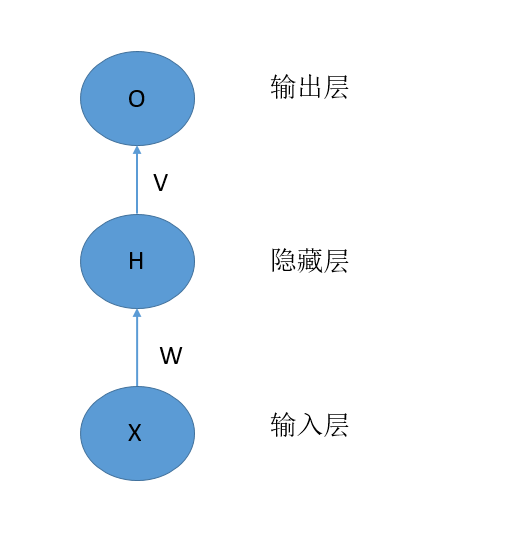X表示输入层的向量，H表示隐藏层的向量，O表示输出层的向量。W表示从输入层到隐藏层的权重矩阵，V表示从隐藏层到输出层的权重矩阵。其中，每一层可以有多个神经元。每一层神经元的个数代表的是每一层的维度。
我们将该网络展开成神经元的形式如下：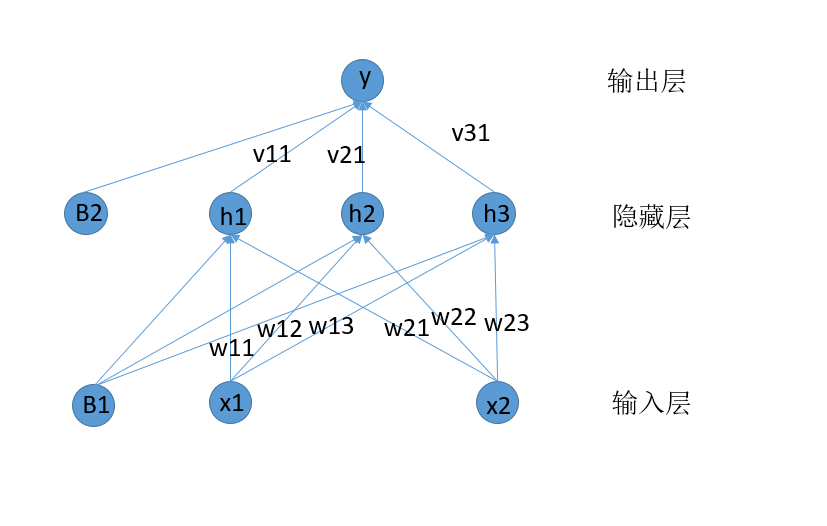我们可以看到的是，对于每一个隐藏层的神经元，其结果是由输入层的向量和权重W来决定的。似乎是有这样的一种可能，每一个隐藏层的神经元是和其排列在前面的神经元之间是有关系的，也就是排列在前面隐藏层的神经元也作为当前隐藏层神经元的一个输入。这就引出来了循环神经网络。
我们先给出循环神经网络的基本结构：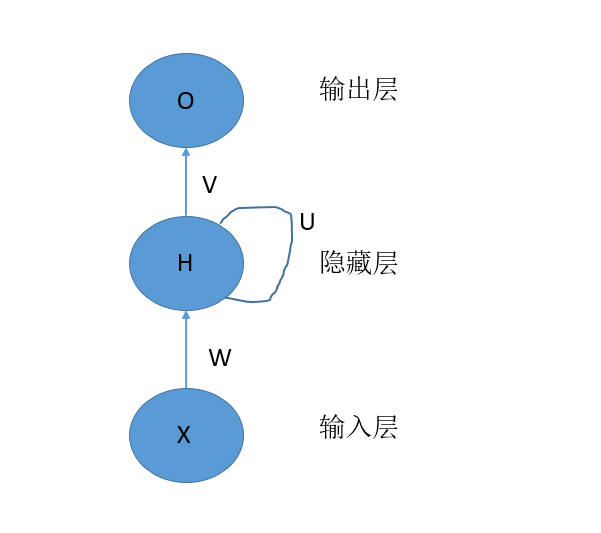在上面的结构中，我们能够发现，对于隐藏层的神经元，其中有一个自我传递信息的过程，下面是具体的展开图：可以理解为，网络的输入通过时间进行向后传播，每次输入一个X，产生一个St和一个O，然后下一个时刻的X和前一个时刻的H作为当前时刻的输入，其中X通过W来控制权重，H通过U来控制权重。我们将上述的神经网络结构成为：单向循环神经网络。
在对于该神经网络进行进一步的展开(这里，我们只展开一个时刻的神经单元)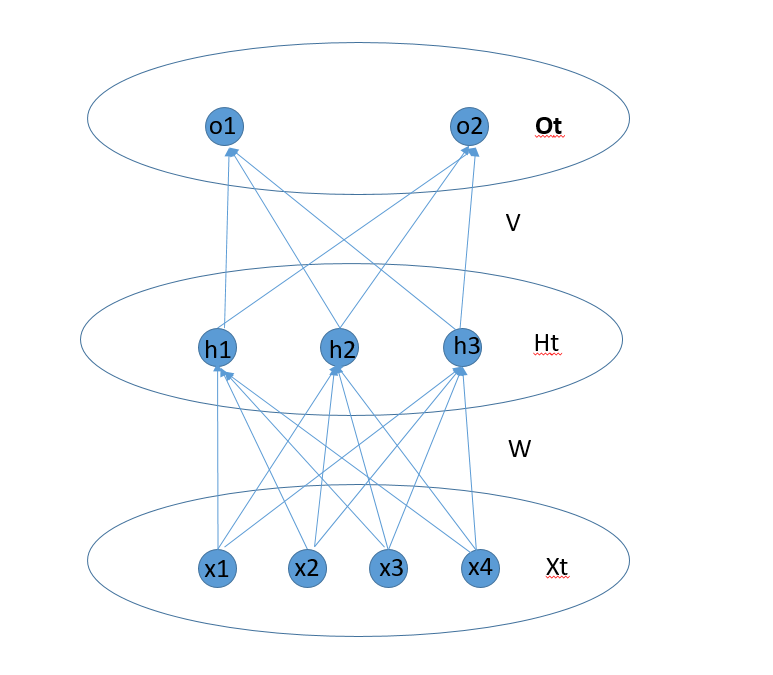2 BPTT算法以及前向和后向传播算法
2.1 前向传播过程

从输入层神经元到隐藏层神经元。
从隐藏层神经元到隐藏层神经元。
隐藏层神经元整合两个部分的输入。
从隐藏层神经元到输出层神经元。

2.1.1 从输入层神经元到隐藏层神经元
这个部分的前向传播主要是输入神经元信息和对应的权重矩阵W来决定的。具体的计算公式为：
$W^TX_t$
注意，这个部分中，X是一个向量，W是一个权重矩阵，通过W来将X转换成另外的向量。
2.1.2 从隐藏层神经元到隐藏层神经元
这个部分主要是前一个时刻的隐藏层神经元向当前时刻的神经元的信息传递，其值有$S_{t-1}$和对应的权重矩阵U来决定。公式如下：
$U^TS_{t-1}$
注意，这个部分中，$S_{t-1}$是一个向量，U是一个权重矩阵，通过U来将$S_{t-1}$转换成另外的向量。
2.1.3 隐藏层神经元整合两个部分的输入
这一部分，主要是当前时刻的神经元$h_t$，将两个部分的输入整合，激活生成当前时刻隐藏层神经元的输出。
整合的过程就是向量相加，对应的公式为：
$W^TX_t+U^TS_{t-1}$
在假设激活函数为f，则激活后生成当前时刻神经元的值：
$S_t = f(W^TX_t+U^TS_{t-1})$
2.1.4 从隐藏层神经元到输出层神经元
这一部分的信息传递主要是将当前时刻隐藏层神经元的值传递到当前时刻的输出神经元中去。计算公式为：
$O_t = g(V^TS_t)$
其中g表示的也是激活函数。
2.2 前向过程总结
前向过程相对来说比较简单，用两个公式就可以总结其基本的传播过程：
$S_t = f(W^TX_t+U^TS_{t-1})$
$O_t = g(V^TS_t)=g(V^Tf(W^TX_t+U^TS_{t-1}))$
2.3 参数权重说明：
在整个神经网络中，一共包含的三中权重矩阵，第一个矩阵是W，给矩阵的维度为(N,K)，N表示隐藏层神经元值的向量维度，K表示输入神经单元的向量的维度。第二个权重矩阵是V，该矩阵的维度为(L,N)，其中P表示输出层的神经元的向量维度。第三个是权重矩阵U，该矩阵的维度是(N,N)。
2.4 反向传播过程
2.4.1 误差函数
关于误差函数的选择，可以根据具体的任务进行，我们这里选择MSE作为误差计算函数：
$J=\frac{1}{2}∑_{t=1}^T||R_t-O_t||^2$
注意，我们这里在算误差的时候，$R_t和O_t$对应的是t时刻真实标签向量是预测输出的向量，所有采用的了取模计算的|| ||。
将上面的式子展开后得到
$J = \frac{1}{2}∑_{t=1}^T∑_{j=1}^L(R_t(j)-O_t(j))^2$
L表示输入向量的属性值的个数。
2.4.2 反向传播过程
对于最后一个时刻的节点$X_T,H_T,O_T$，我们首先计算这一个时刻的相关误差。我们在这里设置两个临时函数：
$neto_t = V^Tf(W^TX_t+U^TS_{t-1})$
$neth_t = W^TX_t+U^TS_{t-1}$
其中$net_o(t)$表示t时刻没有激活的输出层的向量值，$net_h(t)$表示t时刻没有激活的隐藏层的向量值。
为了清晰，我们再之前单个神经元中的结构拿过来：根据公式和图示，我们能够知道$neth(t)$，表示的是t时刻$[h_1,h_2,h_3]$组成的没有激活时的向量，$neto(t)$表示的是没有激活的$[o_1,o_2]$构成的向量。

对于最后时刻T，我们计算出来的误差是J，首先我们计算：
$\frac{∂J}{∂neto_1(T)}=\frac{∂J}{∂O_T(1)}\frac{∂O_T(1)}{∂neto_T(1)}=(R_T(1)-O_T(1))g'(neto_T(1))$
整理一下有：
$δ_T^O(j)=\frac{∂J}{∂neto_T(j)}=\frac{∂J}{∂O_T(j)}\frac{∂O_T(j)}{∂neto_T(j)}=(R_T(j)-O_T(j))g'(neto_T(j)) 其中j∈[1,L]$

进一步，转换成矩阵的形式：
$δ_T^O=\frac{∂J}{∂neto_T}=\frac{∂J}{∂O_T}\frac{∂O_T}{∂neto_T}=(R_T-O_T)*g'(neto_T) 公式1$

然后我们计算
$\frac{∂J}{∂neth_T(1)}=\frac{∂J}{∂O_T(1)}\frac{∂O_T(1)}{∂neto_T(1)}\frac{∂neto_T(1)}{∂h_T(1)}\frac{∂h_T(1)}{∂neth_T(1)}+\frac{∂J}{∂O_T(2)}\frac{∂O_T(2)}{∂neto_T(2)}\frac{∂neto_T(2)}{∂h_T(1)}\frac{∂h_T(2)}{∂neth_T(1)}$